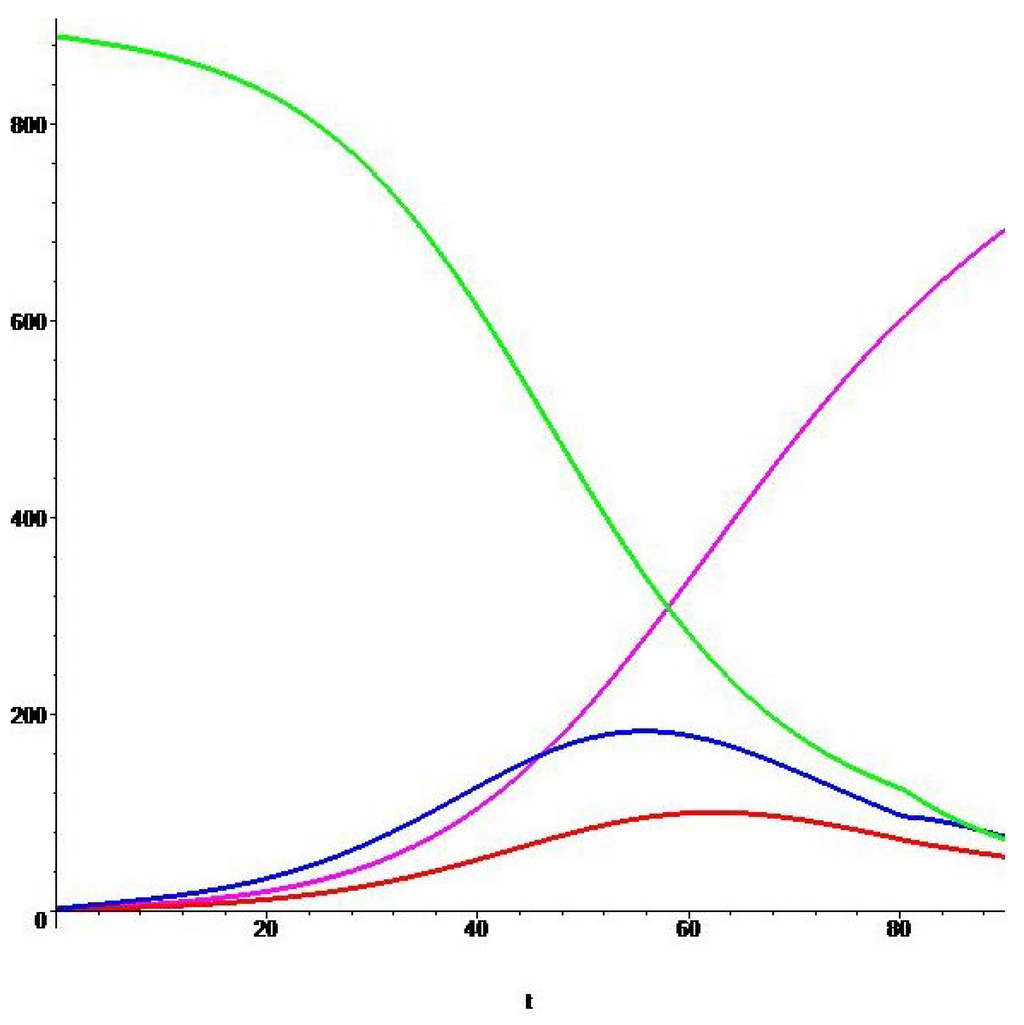Next Article in Journal
Gauge Invariance and Symmetry Breaking by Topology and Energy Gap
Next Article in Special Issue
The San Francisco MSM Epidemic: A Retrospective Analysis
Previous Article in Journal
Reformulated First Zagreb Index of Some Graph Operations
Previous Article in Special Issue
Understanding Visceral Leishmaniasis Disease Transmission and its Control—A Study Based on Mathematical Modeling
Open AccessArticle

# Optimal Intervention Strategies for a SEIR Control Model of Ebola Epidemics

byEllina V. Grigorieva 1,* andEvgenii N. Khailov 2
1
Department of Mathematics and Computer Sciences, Texas Woman’s University, Denton, TX 76204, USA
2
Faculty of Computational Mathematics and Cybernetics, Moscow State Lomonosov University, Moscow 119992, Russia
*
Author to whom correspondence should be addressed.
Mathematics 2015, 3(4), 961-983; https://doi.org/10.3390/math3040961
Received: 30 July 2015 / Revised: 16 September 2015 / Accepted: 10 October 2015 / Published: 21 October 2015
(This article belongs to the Special Issue Optimal Control and Management of Infectious Diseases)
A SEIR control model describing the Ebola epidemic in a population of a constant size is considered over a given time interval. It contains two intervention control functions reflecting efforts to protect susceptible individuals from infected and exposed individuals. For this model, the problem of minimizing the weighted sum of total fractions of infected and exposed individuals and total costs of intervention control constraints at a given time interval is stated. For the analysis of the corresponding optimal controls, the Pontryagin maximum principle is used. According to it, these controls are bang-bang, and are determined using the same switching function. A linear non-autonomous system of differential equations, to which this function satisfies together with its corresponding auxiliary functions, is found. In order to estimate the number of zeroes of the switching function, the matrix of the linear non-autonomous system is transformed to an upper triangular form on the entire time interval and the generalized Rolle’s theorem is applied to the converted system of differential equations. It is found that the optimal controls of the original problem have at most two switchings. This fact allows the reduction of the original complex optimal control problem to the solution of a much simpler problem of conditional minimization of a function of two variables. Results of the numerical solution to this problem and their detailed analysis are provided. View Full-Text
Show FiguresFigure 1

MDPI and ACS Style

Grigorieva, E.V.; Khailov, E.N. Optimal Intervention Strategies for a SEIR Control Model of Ebola Epidemics. Mathematics 2015, 3, 961-983.